tensorflow2_tensorflow2.0 - CSDN

• 2版本教学，比起TensorFlow 1.x版本难度降低了约50%。 3. 案例为师，实战护航 基于计算机视觉和NLP领域的经典数据集，从零开始结合TensorFlow 2与深度学习算法完成多个案例实战。 4. 持续更新，永久...深度学习 深度学习框架 深度学习算法 python深度学习 深度强化学习
• 作者：叶 虎 编辑：陈人和 前 言TensorFlow虽是深度学习领域最广泛使用的框架，但是对比PyTorch这一动态...作者：叶  虎            编辑：陈人和           前  言TensorFlow虽是深度学习领域最广泛使用的框架，但是对比PyTorch这一动态图框架，采用静态图（Graph模式）的TensorFlow确实是难用。好在最近TensorFlow支持了eager模式，对标PyTorch的动态执行机制。更进一步地，Google在最近推出了全新的版本TensorFlow 2.0，2.0版本相比1.0版本不是简单地更新，而是一次重大升级（虽然目前只发布了preview版本）。简单地来说，TensorFlow 2.0默认采用eager执行模式，而且重整了很多混乱的模块。毫无疑问，2.0版本将会逐渐替换1.0版本，所以很有必要趁早入手TensorFlow 2.0。这篇文章将简明扼要地介绍TensorFlow 2.0，以求快速入门。章节目录Eager执行AutoGraph性能优化：tf.function01Eager执行TensorFlow的Eager执行时一种命令式编程（imperative programming），这和原生Python是一致的，当你执行某个操作时是立即返回结果的。而TensorFlow一直是采用Graph模式，即先构建一个计算图，然后需要开启Session，喂进实际的数据才真正执行得到结果。显然，eager执行更简洁，我们可以更容易debug自己的代码，这也是为什么PyTorch更简单好用的原因。一个简单的例子如下：x = tf.ones((2, 2), dtype=tf.dtypes.float32)
y = tf.constant([[1, 2],
[3, 4]], dtype=tf.dtypes.float32)
z = tf.matmul(x, y)
print(z)
# tf.Tensor(
# [[4. 6.]
#  [4. 6.]], shape=(2, 2), dtype=float32)
print(z.numpy())
# [[4. 6.]
# [4. 6.]]可以看到在eager执行下，每个操作后的返回值是tf.Tensor，其包含具体值，不再像Graph模式下那样只是一个计算图节点的符号句柄。由于可以立即看到结果，这非常有助于程序debug。更进一步地，调用tf.Tensor.numpy()方法可以获得Tensor所对应的numpy数组。这种eager执行的另外一个好处是可以使用Python原生功能，比如下面的条件判断：random_value = tf.random.uniform([], 0, 1)
x = tf.reshape(tf.range(0, 4), [2, 2])
print(random_value)
if random_value.numpy() > 0.5:
y = tf.matmul(x, x)
else:
y = tf.add(x, x)这种动态控制流主要得益于eager执行得到Tensor可以取出numpy值，这避免了使用Graph模式下的tf.cond和tf.while等算子。另外一个重要的问题，在egaer模式下如何计算梯度。在Graph模式时，我们在构建模型前向图时，同时也会构建梯度图，这样实际喂数据执行时可以很方便计算梯度。但是eager执行是动态的，这就需要每一次执行都要记录这些操作以计算梯度，这是通过tf.GradientTape来追踪所执行的操作以计算梯度，下面是一个计算实例：w = tf.Variable([[1.0]])
loss = w * w + 2. * w + 5.
print(grad)  # => tf.Tensor([[ 4.]], shape=(1, 1), dtype=float32)对于eager执行，每个tape会记录当前所执行的操作，这个tape只对当前计算有效，并计算相应的梯度。PyTorch也是动态图模式，但是与TensorFlow不同，它是每个需要计算Tensor会拥有grad_fn以追踪历史操作的梯度。TensorFlow 2.0引入的eager提高了代码的简洁性，而且更容易debug。但是对于性能来说，eager执行相比Graph模式会有一定的损失。这不难理解，毕竟原生的Graph模式是先构建好静态图，然后才真正执行。这对于在分布式训练、性能优化和生产部署方面具有优势。但是好在，TensorFlow 2.0引入了tf.function和AutoGraph来缩小eager执行和Graph模式的性能差距，其核心是将一系列的Python语法转化为高性能的graph操作。02AutoGraphAutoGraph在TensorFlow 1.x已经推出，主要是可以将一些常用的Python代码转化为TensorFlow支持的Graph代码。一个典型的例子是在TensorFlow中我们必须使用tf.while和tf.cond等复杂的算子来实现动态流程控制，但是现在我们可以使用Python原生的for和if等语法写代码，然后采用AutoGraph转化为TensorFlow所支持的代码，如下面的例子:def square_if_positive(x):
if x > 0:
x = x * x
else:
x = 0.0
return x
# eager 模式
print('Eager results: %2.2f, %2.2f' % (square_if_positive(tf.constant(9.0)),
square_if_positive(tf.constant(-9.0))))
# graph 模式
tf_square_if_positive = tf.autograph.to_graph(square_if_positive)
with tf.Graph().as_default():
# The result works like a regular op: takes tensors in, returns tensors.
# You can inspect the graph using tf.get_default_graph().as_graph_def()
g_out1 = tf_square_if_positive(tf.constant( 9.0))
g_out2 = tf_square_if_positive(tf.constant(-9.0))
with tf.compat.v1.Session() as sess:
print('Graph results: %2.2f, %2.2f\n' % (sess.run(g_out1), sess.run(g_out2)))上面我们定义了一个square_if_positive函数，它内部使用的Python的原生的if语法，对于TensorFlow 2.0的eager执行，这是没有问题的。然而这是TensorFlow 1.x所不支持的，但是使用AutoGraph可以将这个函数转为Graph函数，你可以将其看成一个常规TensorFlow op，其可以在Graph模式下运行（tf2 没有Session，这是tf1.x的特性，想使用tf1.x的话需要调用tf.compat.v1）。大家要注意eager模式和Graph模式的差异，尽管结果是一样的，但是Graph模式更高效。 从本质上讲，AutoGraph是将Python代码转为TensorFlow原生的代码，我们可以进一步看到转化后的代码:print(tf.autograph.to_code(square_if_positive))
#################################################
from __future__ import print_function
def tf__square_if_positive(x):
try:
with ag__.function_scope('square_if_positive'):
do_return = False
retval_ = None
cond = ag__.gt(x, 0)
def if_true():
with ag__.function_scope('if_true'):
x_1, = x,
x_1 = x_1 * x_1
return x_1
def if_false():
with ag__.function_scope('if_false'):
x = 0.0
return x
x = ag__.if_stmt(cond, if_true, if_false)
do_return = True
retval_ = x
return retval_
except:
ag__.rewrite_graph_construction_error(ag_source_map__)
tf__square_if_positive.autograph_info__ = {}可以看到AutoGraph转化的代码定义了两个条件函数，然后调用if_stmt op，应该就是类似tf.cond的op。AutoGraph支持很多Python特性，比如循环: def sum_even(items):
s = 0
for c in items:
if c % 2 > 0:
continue
s += c
return s
print('Eager result: %d' % sum_even(tf.constant([10,12,15,20])))
tf_sum_even = tf.autograph.to_graph(sum_even)
with tf.Graph().as_default(), tf.compat.v1.Session() as sess:
print('Graph result: %d\n\n' % sess.run(tf_sum_even(tf.constant([10,12,15,20]))))对于大部分Python特性AutoGraph是支持的，但是其仍然有限制，具体可以见Capabilities and Limitations。链接：https://github.com/tensorflow/tensorflow/blob/master/tensorflow/python/autograph/LIMITATIONS.md此外，要注意的一点是，经过AutoGraph转换的新函数是可以eager模式下执行的，但是性能却并不会比转换前的高，你可以对比：x = tf.constant([10, 12, 15, 20])
print("Eager at orginal code:", timeit.timeit(lambda: sum_even(x), number=100))
print("Eager at autograph code:", timeit.timeit(lambda: tf_sum_even(x), number=100))
with tf.Graph().as_default(), tf.compat.v1.Session() as sess:
graph_op = tf_sum_even(tf.constant([10, 12, 15, 20]))
sess.run(graph_op)  # remove first call
print("Graph at autograph code:", timeit.timeit(lambda: sess.run(graph_op), number=100))
##########################################
Eager at orginal code: 0.05176109499999981
Eager at autograph code: 0.11203173799999977
Graph at autograph code: 0.03418808900000059从结果上看，Graph模式下的执行效率是最高的，原来的代码在eager模式下效率次之，经AutoGraph转换后的代码效率最低。所以，在TensorFlow 2.0，我们一般不会直接使用tf.autograph，因为eager执行下效率没有提升。要真正达到Graph模式下的效率，要依赖tf.function这个更强大的利器。03性能优化：tf.function条件随机场（Conditional Random Field，简称CRF）是一种判别式无向图模型。生成式模型是直接对联合分布进行建模，而判别式模型则是对条件分布进行建模。前面介绍的隐马尔可夫模型和马尔可夫随机场都是生成式模型，而条件随机场是判别式模型。尽管eager执行更简洁，但是Graph模式却是性能更高，为了减少这个性能gap，TensorFlow 2.0引入了tf.function，先给出官方对tf.function的说明:function constructs a callable that executes a TensorFlow graph (tf.Graph) created by tracing the TensorFlow operations in func. This allows the TensorFlow runtime to apply optimizations and exploit parallelism in the computation defined by func.简单来说，就是tf.function可以将一个func中的TensorFlow操作构建为一个Graph，这样在调用时是执行这个Graph，这样计算性能更优。比如下面的例子:def f(x, y):
print(x, y)
return tf.reduce_mean(tf.multiply(x ** 2, 3) + y)
g = tf.function(f)
x = tf.constant([[2.0, 3.0]])
y = tf.constant([[3.0, -2.0]])
# f and g will return the same value, but g will be executed as a
# TensorFlow graph.
assert f(x, y).numpy() == g(x, y).numpy()
# tf.Tensor([[2. 3.]], shape=(1, 2), dtype=float32) tf.Tensor([[ 3. -2.]], shape=(1, 2), dtype=float32)
# Tensor("x:0", shape=(1, 2), dtype=float32) Tensor("y:0", shape=(1, 2), dtype=float32)如上面的例子，被tf.function装饰的函数将以Graph模式执行，可以把它想象一个封装了Graph的TF op，直接调用它也会立即得到Tensor结果，但是其内部是高效执行的。我们在内部打印Tensor时，eager执行会直接打印Tensor的值，而Graph模式打印的是Tensor句柄，其无法调用numpy方法取出值，这和TF 1.x的Graph模式是一致的。由于tf.function装饰的函数是Graph执行，其执行速度一般要比eager模式要快，当Graph包含很多小操作时差距更明显，可以比较下卷积和LSTM的性能差距：import timeit
conv_layer = tf.keras.layers.Conv2D(100, 3)
@tf.function
def conv_fn(image):
return conv_layer(image)
image = tf.zeros([1, 200, 200, 100])
# warm up
conv_layer(image); conv_fn(image)
print("Eager conv:", timeit.timeit(lambda: conv_layer(image), number=10))
print("Function conv:", timeit.timeit(lambda: conv_fn(image), number=10))
# 单纯的卷积差距不是很大
# Eager conv: 0.44013839924952197
# Function conv: 0.3700763391782858
lstm_cell = tf.keras.layers.LSTMCell(10)
@tf.function
def lstm_fn(input, state):
return lstm_cell(input, state)
input = tf.zeros([10, 10])
state = [tf.zeros([10, 10])] * 2
# warm up
lstm_cell(input, state); lstm_fn(input, state)
print("eager lstm:", timeit.timeit(lambda: lstm_cell(input, state), number=10))
print("function lstm:", timeit.timeit(lambda: lstm_fn(input, state), number=10))
# 对于LSTM比较heavy的计算，Graph执行要快很多
# eager lstm: 0.025562446062237565
# function lstm: 0.0035498656569271647要想灵活使用tf.function，必须深入理解它背后的机理，这里简单地谈一下。在TF 1.x时，首先要创建静态计算图，然后新建Session真正执行不同的运算：import tensorflow as tf
x = tf.placeholder(tf.float32)
y = tf.square(x)
sess = tf.Session()
z0 = sess.run([z], feed_dict={x: 2.})        # 6.0
z1 = sess.run([z], feed_dict={x: 2., y: 2.}) # 4.0尽管上面只定义了一个graph，但是两次不同的sess执行（运行时）其实是执行两个不同的程序或者说subgraph：def compute_z0(x):
def compute_z1(x, y):
return tf.add(x,  y)这里我们将两个不同的subgraph封装到了两个python函数中。更进一步地，我们可以不再需要Session，当执行这两个函数时，直接调用对应的计算图就可以，这就是tf.function的功效：import tensorflow as tf
@tf.function
def compute_z1(x, y):
@tf.function
def compute_z0(x):
return compute_z1(x, tf.square(x))
z0 = compute_z0(2.)
z1 = compute_z1(2., 2.)可以说tf.function内部管理了一系列Graph，并控制了Graph的执行。另外一个问题时，虽然函数内部定义了一系列的操作，但是对于不同的输入，是需要不同的计算图。如函数的输入Tensor的shape或者dtype不同，那么计算图是不同的，好在tf.function支持这种多态性（polymorphism）# Functions are polymorphic
@tf.function
def double(a):
print("Tracing with", a)
return a + a
print(double(tf.constant(1)))
print(double(tf.constant(1.1)))
print(double(tf.constant([1, 2])))
# Tracing with Tensor("a:0", shape=(), dtype=int32)
# tf.Tensor(2, shape=(), dtype=int32)
# Tracing with Tensor("a:0", shape=(), dtype=float32)
# tf.Tensor(2.2, shape=(), dtype=float32)
# Tracing with Tensor("a:0", shape=(2,), dtype=int32)
# tf.Tensor([2 4], shape=(2,), dtype=int32)注意函数内部的打印，当输入tensor的shape或者类型发生变化，打印的东西也是相应改变。所以，它们的计算图（静态的）并不一样。tf.function这种多态特性其实是背后追踪了（tracing）不同的计算图。具体来说，被tf.function装饰的函数f接受一定的Tensors，并返回0到任意到Tensor，当装饰后的函数F被执行时：根据输入Tensors的shape和dtypes确定一个"trace_cache_key"；每个"trace_cache_key"映射了一个Graph，当新的"trace_cache_key"要建立时，f将构建一个新的Graph，若"trace_cache_key"已经存在，那么直需要从缓存中查找已有的Graph即可；将输入Tensors喂进这个Graph，然后执行得到输出Tensors。这种多态性是我们需要的，因为有时候我们希望输入不同shape或者dtype的Tensors，但是当"trace_cache_key"越来越多时，意味着你要cache了庞大的Graph，这点是要注意的。另外，tf.function提供了input_signature，这个参数采用tf.TensorSpec指定了输入到函数的Tensor的shape和dtypes，如下面的例子：@tf.function(input_signature=[tf.TensorSpec(shape=None, dtype=tf.float32)])
def f(x):
print(f(tf.constant(1.0)))  # tf.Tensor(2.0, shape=(), dtype=float32)
print(f(tf.constant([1.0,]))) # tf.Tensor([2.], shape=(1,), dtype=float32)
print(f(tf.constant()))  # ValueError: Python inputs incompatible with input_signature此时，输入Tensor的dtype必须是float32，但是shape不限制，当类型不匹配时会出错。tf.function的另外一个参数是autograph，默认是True，意思是在构建Graph时将自动使用AutoGraph，这样你可以在函数内部使用Python原生的条件判断以及循环语句，因为它们会被tf.cond和tf.while_loop转化为Graph代码。注意的一点是判断分支和循环必须依赖于Tensors才会被转化，当autograph为False时，如果存在判断分支和循环必须依赖于Tensors的情况将会出错。如下面的例子。def sum_even(items):
s = 0
for c in items:
if c % 2 > 0:
continue
s += c
return s
sum_even_autograph_on = tf.function(sum_even, autograph=True)
sum_even_autograph_off = tf.function(sum_even, autograph=False)
x = tf.constant([10, 12, 15, 20])
sum_even(x) # OK
sum_even_autograph_on(x) # OK
sum_even_autograph_off(x) # TypeError: Tensor objects are only iterable when eager execution is enabled很容易理解，应用tf.function之后是Graph模式，Tensors是不能被遍历的，但是采用AutoGraph可以将其转换为Graph代码，所以可以成功。大部分情况，我们还是默认开启autograph。最要的是tf.function可以应用到类方法中，并且可以引用tf.Variable，可以看下面的例子：class ScalarModel(object):
def __init__(self):
self.v = tf.Variable(0)
@tf.function
def increment(self, amount):
model1 = ScalarModel()
model1.increment(tf.constant(3))
assert int(model1.v) == 3
model1.increment(tf.constant(4))
assert int(model1.v) == 7
model2 = ScalarModel()  # model1和model2 拥有不同变量
model2.increment(tf.constant(5))
assert int(model2.v) == 5后面会讲到，这个特性可以应用到tf.Keras的模型构建中。上面这个例子还有一点，就是可以在function中使用tf.assign这类具有副作用（改变Variable的值）的操作，这对于模型训练比较重要。前面说过，python原生的print函数只会在构建Graph时打印一次Tensor句柄。如果想要打印Tensor的具体值，要使用tf.print：@tf.function
def print_element(items):
for c in items:
tf.print(c)
x = tf.constant([1, 5, 6, 8, 3])
print_element(x)这里就对tf.function做这些介绍，但是实际上其还有更多复杂的使用须知，详情可以参考TensorFlow 2.0: Functions, not Sessions。链接：https://github.com/tensorflow/community/blob/master/rfcs/20180918-functions-not-sessions-20.md参考：TensorFlow官网：https://tensorflow.google.cn/versions/r2.0/api_docs/END往期回顾【1】network sliming:加快模型速度同时不损失精度【2】理解Batch Normalization（含实现代码）【3】图像降维之Isomap特征抽取方法【4】语义分割网络经典：FCN与SegNet【5】One Day One GAN [DAY 4]机器学习算法工程师                            一个用心的公众号长按，识别，加关注进群，学习，得帮助你的关注，我们的热度，我们一定给你学习最大的帮助你点的每个赞，我都认真当成了喜欢


展开全文• 【2019年好评如潮的《玩转TensorFlow 2》重磅更新】2020年1月发布【TensorFlow 2.1】，进一步增强了Keras API。本课程带您全面了解TensorFlow API，掌握【Keras API】用法，并通过丰富的【实战案例】示范TensorFlow...深度学习 深度学习框架 深度学习算法 python深度学习 深度强化学习
• 【导读】 TensorFlow 2.0 于近期正式发布后，立即受到学术界与科研界的广泛关注与好评。此前，AI 科技大本营曾特邀专家回顾了 TensorFlow 2.0 从初版到最新版本的发展史。今天，我们将介绍一本全面详解 TensorFlow ...【导读】 TensorFlow 2.0 于近期正式发布后，立即受到学术界与科研界的广泛关注与好评。此前，AI 科技大本营曾特邀专家回顾了 TensorFlow 2.0 从初版到最新版本的发展史。今天，我们将介绍一本全面详解 TensorFlow 2.0 的手册。这本手册是 Google Summer of Code 2019 项目之一，从基础安装与环境配置、部署，到大规模训练与加速、扩展，全方位讲解 TensorFlow 2.0  的入门要点，并附录相关资料供读者参考。

这是一本简明的 TensorFlow 2.0 入门指导手册，基于 Keras 和 Eager Execution（即时运行）模式，力图让具备一定机器学习及 Python 基础的开发者们快速上手 TensorFlow 2.0。

本手册的所有代码基于 TensorFlow 2.0 正式版及 beta1 版本。

中文指南：https://tf.wiki/

GitHub链接：https://github.com/snowkylin/tensorflow-handbook

文中的所有示例代码：https://github.com/snowkylin/tensorflow-handbook/tree/master/source/_static/code/zh

本手册所有章节如下图，不仅包含 TensorFlow 的概述，还含有基础、部署、大规模训练与加速、扩展，以及附录部分。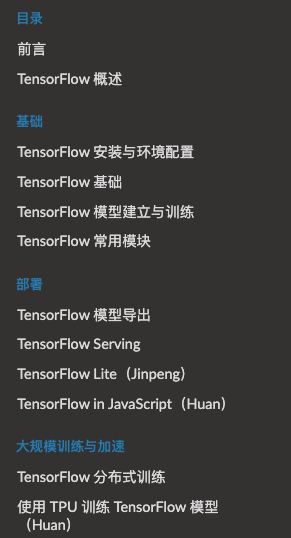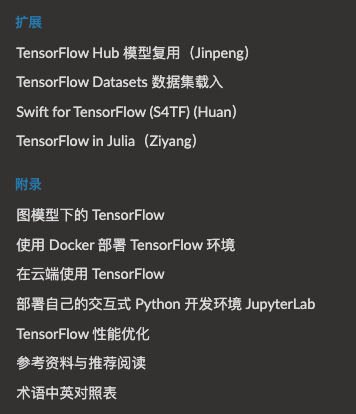前言

此前，TensorFlow 所基于的传统 Graph Execution 的弊端，如入门门槛高、调试困难、灵活性差、无法使用 Python 原生控制语句等早已被开发者诟病许久。一些新的基于动态图机制的深度学习框架（如 PyTorch）也横空出世，并以其易用性和快速开发的特性而占据了一席之地。尤其是在学术研究等需要快速迭代模型的领域，PyTorch 等新兴深度学习框架已经成为主流。作者所在的数十人的机器学习实验室中，竟只有其一人 “守旧” 地使用 TensorFlow。

然而，直到目前，市面上相关的 TensorFlow 相关的中文技术书籍及资料仍然基于传统的 Graph Execution 模式，让不少初学者（尤其是刚学过机器学习课程的大学生）望而却步。由此，在 TensorFlow 正式支持 Eager Execution 之际，有必要出现一本全新的技术手册，帮助初学者及需要快速迭代模型的研究者，以一个全新的角度快速入门 TensorFlow。

同时，本手册还有第二个任务。市面上与 TensorFlow 相关的中文技术书籍大都以深度学习为主线，将 TensorFlow 作为这些深度学习模型的实现方式。这样固然有体系完整的优点，然而对于已经对机器学习或深度学习理论有所了解，希望侧重于学习 TensorFlow 本身的读者而言，就显得不够友好。

于是，作者希望编写一本手册，以尽量精简的篇幅展示 TensorFlow 作为一个计算框架的主要特性，并弥补官方手册的不足，力图能让已经有一定机器学习 / 深度学习知识及编程能力的读者迅速上手 TensorFlow，并在实际编程过程中可以随时查阅并解决实际问题。

适用群体

本书适用于以下读者：

已有一定机器学习 / 深度学习基础，希望将所学理论知识使用 TensorFlow 进行具体实现的学生和研究者；

曾使用或正在使用 TensorFlow 1.X 版本或其他深度学习框架（比如 PyTorch），希望了解 TensorFlow 2.0 新特性的开发者；

希望将已有的 TensorFlow 模型应用于业界的开发者或工程师。

提示

本书不是一本机器学习 / 深度学习原理入门手册。若希望进行机器学习 / 深度学习理论的入门学习，可参考附录中提供的一些入门资料。

TensorFlow 概述

学生和研究者：模型的建立与训练

开发者和工程师：模型的调用与部署

TensorFlow 能帮助我们做什么？

基础

TensorFlow 安装与环境配置

TensorFlow 的最新安装步骤可参考官方网站上的说明（https://tensorflow.google.cn/install）。TensorFlow 支持 Python、Java、Go、C 等多种编程语言以及 Windows、OSX、Linux 等多种操作系统，此处及后文均以 Python 3.7 为准。

提示

本章介绍在一般的个人电脑或服务器上直接安装 TensorFlow 2.0 的方法。关于在容器环境（Docker）、云平台中部署 TensorFlow 或在线上环境中使用 TensorFlow 的方法，见附录 使用 Docker 部署 TensorFlow 环境和在云端使用 TensorFlow 。软件的安装方法往往具有时效性，本节的更新日期为 2019 年 10 月。

一般安装步骤

GPU 版本 TensorFlow 安装指南

GPU 硬件的准备

NVIDIA 驱动程序的安装

CUDA Toolkit 和 cnDNN 的安装

第一个程序

IDE 设置

TensorFlow 所需的硬件配置 *

TensorFlow 基础

本章介绍 TensorFlow 的基本操作。

TensorFlow 1+1

自动求导机制

基础示例：线性回归

NumPy 下的线性回归

TensorFlow 下的线性回归

TensorFlow 模型建立与训练

本章介绍如何使用 TensorFlow 快速搭建动态模型。

模型（Model）与层（Layer）

基础示例：多层感知机（MLP）

数据获取及预处理：tf.keras.datasets

模型的构建：tf.keras.Model 和 tf.keras.layers

模型的训练：tf.keras.losses 和 tf.keras.optimizer

模型的评估：tf.keras.metrics

卷积神经网络（CNN）

使用 Keras 实现卷积神经网络

使用 Keras 中预定义的经典卷积神经网络结构

循环神经网络（RNN）

深度强化学习（DRL）

Keras Pipeline *

Keras Sequential/Functional API 模式建立模型

使用 Keras Model 的 compile 、 fit 和 evaluate 方法训练和评估模型

自定义层、损失函数和评估指标 *

自定义层

自定义损失函数和评估指标

TensorFlow 常用模块

tf.train.Checkpoint ：变量的保存与恢复

TensorBoard：训练过程可视化

tf.data ：数据集的构建与预处理

数据集对象的建立

数据集对象的预处理

数据集元素的获取与使用

实例：cats_vs_dogs 图像分类

@tf.function ：Graph Execution 模式 *

@tf.function 基础使用方法

@tf.function 内在机制

AutoGraph：将 Python 控制流转换为 TensorFlow 计算图

使用传统的 tf.Session

tf.TensorArray ：TensorFlow 动态数组 *

tf.config：GPU 的使用与分配 *

指定当前程序使用的 GPU

设置显存使用策略

单 GPU 模拟多 GPU 环境

部署

TensorFlow 模型导出

使用 SavedModel 完整导出模型

Keras Sequential save 方法（Jinpeng）

TensorFlow Serving

TensorFlow Serving 安装

TensorFlow Serving 模型部署

Keras Sequential 模式模型的部署

自定义 Keras 模型的部署

在客户端调用以 TensorFlow Serving 部署的模型

Python 客户端示例

Node.js 客户端示例（Ziyang）

TensorFlow Lite（Jinpeng）

模型转换

Android 部署

Quantization 模型转换

总结

TensorFlow in JavaScript（Huan）

TensorFlow.js 简介

浏览器中使用 TensorFlow.js 的优势

TensorFlow.js 性能对比

TensorFlow.js 环境配置

在浏览器中使用 TensorFlow.js

在 Node.js 中使用 TensorFlow.js

在微信小程序中使用 TensorFlow.js

TensorFlow.js 模型部署

通过 TensorFlow.js 加载 Python 模型

使用 TensorFlow.js 模型库

TensorFlow.js 模型训练 *

大规模训练与加速

TensorFlow 分布式训练

当我们拥有大量计算资源时，通过使用合适的分布式策略，可以充分利用这些计算资源，从而大幅压缩模型训练的时间。针对不同的使用场景，TensorFlow 在 tf.distribute.Strategy 中为我们提供了若干种分布式策略，从而能够更高效地训练模型。

单机多卡训练：MirroredStrategy

多机训练：MultiWorkerMirroredStrategy

使用 TPU 训练 TensorFlow 模型（Huan）

2017 年 5 月，Alpha Go 在中国乌镇围棋峰会上，与世界第一棋士柯洁比试，并取得了三比零全胜战绩。之后的版本 Alpha Zero 可以通过自我学习 21 天即可以达到胜过中国顶尖棋手柯洁的 Alpha Go Master 的水平。

Alpha Go 背后的动力全部由 TPU 提供，TPU 使其能够更快地 “思考” 并在每一步之间看得更远。

TPU 简介

什么是 TPU

为什么使用 TPU

TPU 性能

TPU 环境配置

Cloud TPU

TPU 基础使用

扩展

TensorFlow Hub 模型复用（Jinpeng）

TensorFlow Datasets 数据集载入

Swift for TensorFlow (S4TF) (Huan）

S4TF 简介

为什么要使用 Swift 进行 Tensorflow 开发

S4TF 环境配置

本地安装 Swift for Tensorflow

在 Colaboratory 中快速体验 Swift for Tensorflow

在 Docker 中快速体验 Swift for TensorFlow

S4TF 基础使用

在 Swift 中使用标准的 TensorFlow API

在 Swift 中直接加载 Python 语言库

语言原生支持自动微分

MNIST 数字分类

TensorFlow in Julia（Ziyang）

TensorFlow.jl 简介

为什么要使用 Julia ？

TensorFlow.jl 环境配置

在 docker 中快速体验 TensorFlow.jl

在 julia 包管理器中安装 TensorFlow.jl

TensorFlow.jl 基础使用

MNIST 数字分类

附录

图模型下的 TensorFlow

TensorFlow 1+1

基础示例：线性回归

使用 Docker 部署 TensorFlow 环境

在云端使用 TensorFlow

在 Colab 中使用 TensorFlow

在 Compute Engine 建立带 GPU 的实例并部署 TensorFlow

使用 AI Platform 中的 Notebook 建立带 GPU 的在线 JupyterLab 环境

在阿里云上使用 GPU 实例运行 Tensorflow（Ziyang）

部署自己的交互式 Python 开发环境 JupyterLab

TensorFlow 性能优化

参考资料与推荐阅读

术语中英对照表

答疑区

（中文）TensorFlow 中文社区 “简单粗暴 TensorFlow” 版面：https://www.tensorflowers.cn/b/48 （中文的疑问和建议请来此处，将以中文回答和讨论。欢迎使用中文的开发者们前来 TensorFlow 中文社区交流讨论）

（英文）https://github.com/snowkylin/tensorflow-handbook/releases （英文的疑问或建议可在 GitHub issue 中提出，会以英文回答）

PDF 下载（旧版）：

中文版：https://www.tensorflowers.cn/t/6230 （同时也有英文版下载）

英文版：https://github.com/snowkylin/tensorflow-handbook/releases

搜索页面：https://tf.wiki/search.html

作者乃北大学霸

最后，我们有必要介绍一下《简单粗暴TensorFlow 2.0》的作者李锡涵。（图片来源：领英）

据领英资料显示，李锡涵是来自北大信息科学技术学院的准理科硕士生，担任见习顾问和研究助理，2016年本科毕业于浙大竺可桢学院，并在 2015 年以交换生的身份进入交通大学。


展开全文• 但是老版的tensorflow程序在新版本中几乎都无法继续使用，所以我们有必要学习新版tensorflow2的新用法。 课程介绍： 我们的这门课程适合小白学习，也适合有基础的同学学习。课程会从0开始学习，...深度学习 深度学习框架 深度学习算法 python深度学习 深度强化学习
• ## 使用TensorFlow实现二分类

万次阅读 热门讨论 2018-09-21 08:20:48
使用TensorFlow构建一个神经网络来实现二分类，主要包括输入数据格式、隐藏层数的定义、损失函数的选择、优化函数的选择、输出层。下面通过numpy来随机生成一组数据，通过定义一种正负样本的区别，通过TensorFlow来...
使用TensorFlow构建一个神经网络来实现二分类，主要包括输入数据格式、隐藏层数的定义、损失函数的选择、优化函数的选择、输出层。下面通过numpy来随机生成一组数据，通过定义一种正负样本的区别，通过TensorFlow来构造一个神经网络来实现二分类。

一、神经网络结构

输入数据：定义输入一个二维数组（x1,x2），数据通过numpy来随机产生，将输出定义为0或1，如果x1+x2<1,则y为1，否则y为0。

隐藏层：定义两层隐藏层，隐藏层的参数为(2,3)，两行三列的矩阵，输入数据通过隐藏层之后，输出的数据为(1,3)，t通过矩阵之间的乘法运算可以获得输出数据。

损失函数：使用交叉熵作为神经网络的损失函数，常用的损失函数还有平方差。

输出数据：将隐藏层的输出数据通过（3,1）的参数，输出一个一维向量，值的大小为0或1。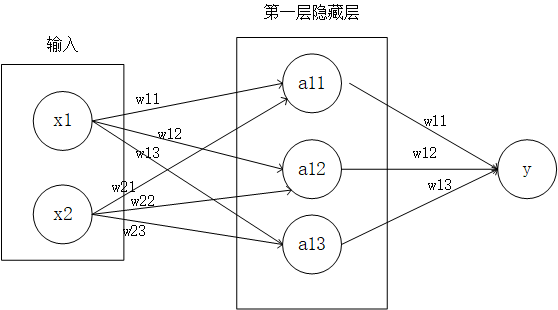二、TensorFlow代码的实现

import tensorflow as tf
from numpy.random import RandomState

if __name__ == "__main__":
#定义每次训练数据batch的大小为8，防止内存溢出
batch_size = 8
#定义神经网络的参数
w1 = tf.Variable(tf.random_normal([2,3],stddev=1,seed=1))
w2 = tf.Variable(tf.random_normal([3,1],stddev=1,seed=1))
#定义输入和输出
x = tf.placeholder(tf.float32,shape=(None,2),name="x-input")
y_ = tf.placeholder(tf.float32,shape=(None,1),name="y-input")
#定义神经网络的前向传播过程
a = tf.matmul(x,w1)
y = tf.matmul(a,w2)
#定义损失函数和反向传播算法
#使用交叉熵作为损失函数
#tf.clip_by_value(t, clip_value_min, clip_value_max,name=None)
#基于min和max对张量t进行截断操作，为了应对梯度爆发或者梯度消失的情况
cross_entropy = -tf.reduce_mean(y_ * tf.log(tf.clip_by_value(y,1e-10,1.0)))
#通过随机函数生成一个模拟数据集
rdm = RandomState(1)
# 定义数据集的大小
dataset_size = 128
# 模拟输入是一个二维数组
X = rdm.rand(dataset_size,2)
#定义输出值，将x1+x2 < 1的输入数据定义为正样本
Y = [[int(x1+x2 < 1)] for (x1,x2) in X]
#创建会话运行TensorFlow程序
with tf.Session() as sess:
#初始化变量  tf.initialize_all_variables()
init = tf.initialize_all_variables()
sess.run(init)
#设置神经网络的迭代次数
steps = 5000
for i in range(steps):
#每次选取batch_size个样本进行训练
start = (i * batch_size) % dataset_size
end = min(start + batch_size,dataset_size)
#通过选取样本训练神经网络并更新参数
sess.run(train_step,feed_dict={x:X[start:end],y_:Y[start:end]})
#每迭代1000次输出一次日志信息
if i % 1000 == 0 :
# 计算所有数据的交叉熵
total_cross_entropy = sess.run(cross_entropy,feed_dict={x:X,y_:Y})
# 输出交叉熵之和
print("After %d training step(s),cross entropy on all data is %g"%(i,total_cross_entropy))
#输出参数w1
print(w1.eval(session=sess))
#输出参数w2
print(w2.eval(session=sess))
'''
After 0 training step(s),cross entropy on all data is 0.0674925
After 1000 training step(s),cross entropy on all data is 0.0163385
After 2000 training step(s),cross entropy on all data is 0.00907547
After 3000 training step(s),cross entropy on all data is 0.00714436
After 4000 training step(s),cross entropy on all data is 0.00578471
[[-1.96182752  2.58235407  1.68203771]
[-3.46817183  1.06982315  2.11788988]]
[[-1.82471502]
[ 2.68546653]
[ 1.41819501]]
'''

--------------------------------2018/9/16更新(非常感谢al_kk的提醒)---------------------------------------

一、为什么说这个损失函数的定义存在问题呢？

上面程序中定义的输入的y的shape为，也就是y的类别为0或1，对于单分类问题交叉熵损失函数的定义应该为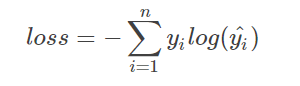其中n为y的种类，在上面的例子中需要包含0和1的y_*log(y)(y_表示真实类别，y表示预测类别)，而上面的例子中只包含了一个y_*log(y)，在上例中正确的损失函数定义应该为loss = y_*log(y) + (1-y_) * log(1-y)。为了便于大家理解，我引用al_kk：“如果只有一个类别的交叉熵即y_ * log(y)，如果真实类别y_为0，那么无论预测值y为任何值的时候，损失函数始终为0”。除此之外，大家可以想一下，当预测值始终为1的时候，那么损失函数是不是就会一直为0，这也是为什么输出预测值y的时候，y的值都是大于1的。如果将y的shape改为的话，就可以使用y_*log(y)。

二、修改之后的二分类程序

import tensorflow as tf
import numpy as np
from numpy.random import RandomState
import matplotlib.pyplot as plt

if __name__ == "__main__":
#定义神经网络的参数
w = tf.Variable(tf.random_normal([2,1],stddev=1,seed=1))
b = tf.Variable(tf.random_normal(,stddev=1,seed=1))
#定义输入和输出
x = tf.placeholder(tf.float32,shape=(None,2),name="x-input")
y_ = tf.placeholder(tf.float32,shape=(None,1),name="y-input")
#定义神经网络的前向传播过程
y = tf.nn.sigmoid(tf.matmul(x,w) + b)
#基于min和max对张量t进行截断操作，为了应对梯度爆发或者梯度消失的情况
cross_entropy = -tf.reduce_mean(y_ * tf.log(tf.clip_by_value(y,1e-10,1.0))+(1-y_) * tf.log(tf.clip_by_value(1-y,1e-10,1.0)))
#通过随机函数生成一个模拟数据集
rdm = RandomState(1)
# 定义数据集的大小
dataset_size = 100
# 模拟输入是一个二维数组
X = rdm.rand(dataset_size,2)
#定义输出值，将x1+x2 < 1的输入数据定义为正样本
Y = [[int(x1+x2 < 1)] for (x1,x2) in X]
#创建会话运行TensorFlow程序
with tf.Session() as sess:
#初始化变量  tf.initialize_all_variables()
init = tf.initialize_all_variables()
sess.run(init)
#设置神经网络的迭代次数
steps = 500
for i in range(steps):
#通过选取样本训练神经网络并更新参数
for (input_x,input_y) in zip(X,Y):
input_x = np.reshape(input_x,(1,2))
input_y = np.reshape(input_y,(1,1))
sess.run(train_step,feed_dict={x:input_x,y_:input_y})
#每迭代1000次输出一次日志信息
if i % 100 == 0:
# 计算所有数据的交叉熵
total_cross_entropy = sess.run(cross_entropy,feed_dict={x:X,y_:Y})
# 输出交叉熵之和
print("After %d training step(s),cross entropy on all data is %g"%(i,total_cross_entropy))
#预测输入X的类别
pred_Y = sess.run(y,feed_dict={x:X})
index = 1
for pred,real in zip(pred_Y,Y):
print(pred,real)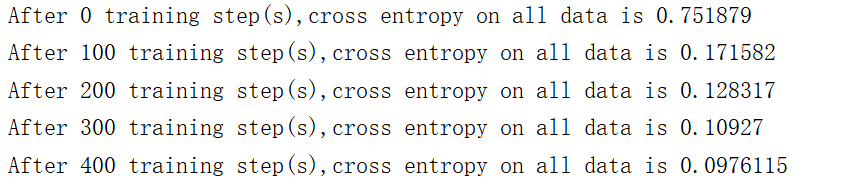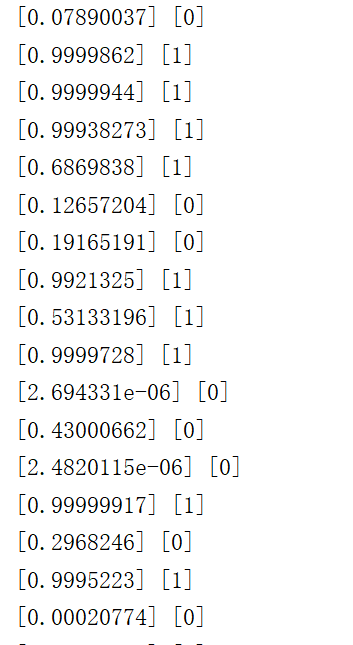展开全文• ## TensorFlow2总结

千次阅读 2019-07-25 19:09:15
Tensor数据类型 list: [1,1.2,'hello'] ，内存占用大，处理速度慢 np.array，数据同类型，但不支持GPU和自动求导 tf.Tensor，为了弥补numpy的缺点，为深度学习而生，支持GPU与自动求导 tensor： ...
Tensor数据类型

list: [1,1.2,'hello'] ，内存占用大，处理速度慢
np.array，数据同类型，但不支持GPU和自动求导
tf.Tensor，为了弥补numpy的缺点，为深度学习而生，支持GPU与自动求导
tensor：
scalar：标量，1.1，dim = 0
vector：向量，[1.1],[1.1,2.2,...]，dim = 1
matrix: 矩阵，[[1.1,2.2],[3.3,4.4]]，dim = 2
tensor：rank>2 ，dim > 2

数据类型：
Int, float, double
bool
string

定义tensor
tf.constant(1)  # 定义int，普通的tensor
tf.constant(1.)  # 定义float
tf.constant([True, False])  # 定义bool
tf.constant('hello nick')   # 定义string

属性

with tf.device('cpu'):
a = tf.constant()   # 在cpu创建
with tf.device('gpu'):
b = tf.constant() # 在gpu创建

a.device # 设备属性
a.gpu()  # cpu转gpu
b.cpu()  # gpu转cpu
a.numpy()# 获取numpy数据类型
a.shape  # 获取a的属性
a.ndim   # 获取维度
tf.rank(a)  # 获取维度
a.name  # 1.+历史遗留问题

数据类型判断

instance(a,tf.Tensor) # 判断是否为tensor （不推荐）
tf.is_tensor(a)  # 判断是否为tensor   （推荐）
a.dtype,b.dtype,c.dtype  # 判断数据类型

数据类型转换

a = np.arange(5)
aa = tf.convert_to_tensor(a,dtype=tf.int32) # numpy转tensor

tf.cast(aa,dtype=tf.float32)  # tensor之间数据类型转换 tf.int32转tf.float32

b = tf.constant([0,1])
tf.cast(b,dtype=tf.bool) # int转bool

# tf.Variable
a = tf.range(5)    # 生成一个tensor
b = tf.Variable(a, name='input_data') # tensor转为Variable后具有求导的特性，即自动记录a的梯度相关信息
b.name      # input_data:0
b.trainable # True  # 可训练

isinstance(b,tf.Tensor)    # False
isinstance(b,tf.Variable)  # True
tf.is_tensor(b)  # True    # 推荐使用

tensor转numpy

a= tf.range(5)
a.numpy()

# a必须是scalar
a = tf.ones([])
a.numpy()
int(a)
float(a)

创建Tensor

from numpy, list
* zeros, ones, fill
* random  # if big dimension, random initial
* constant
* Application

numpy, list

numpy

import numpy as np
import tensorflow as tf

tf.convert_to_tensor(np.ones([2, 3]))

<tf.Tensor: id=0, shape=(2, 3), dtype=float64, numpy=
array([[1., 1., 1.],
[1., 1., 1.]])>

tf.convert_to_tensor(np.zeros([2, 3]))

<tf.Tensor: id=2, shape=(2, 3), dtype=float64, numpy=
array([[0., 0., 0.],
[0., 0., 0.]])>

list

tf.convert_to_tensor([1, 2])

<tf.Tensor: id=4, shape=(2,), dtype=int32, numpy=array([1, 2], dtype=int32)>

tf.convert_to_tensor([1, 2.])

<tf.Tensor: id=6, shape=(2,), dtype=float32, numpy=array([1., 2.], dtype=float32)>

tf.convert_to_tensor([, [2.]])

<tf.Tensor: id=8, shape=(2, 1), dtype=float32, numpy=
array([[1.],
[2.]], dtype=float32)>

zeros, ones, fill

zeros

tf.zeros([])

<tf.Tensor: id=10, shape=(), dtype=float32, numpy=0.0>

tf.zeros()

<tf.Tensor: id=14, shape=(1,), dtype=float32, numpy=array([0.], dtype=float32)>

tf.zeros([2, 2])

<tf.Tensor: id=18, shape=(2, 2), dtype=float32, numpy=
array([[0., 0.],
[0., 0.]], dtype=float32)>

tf.zeros([2, 3, 3])

<tf.Tensor: id=22, shape=(2, 3, 3), dtype=float32, numpy=
array([[[0., 0., 0.],
[0., 0., 0.],
[0., 0., 0.]],

[[0., 0., 0.],
[0., 0., 0.],
[0., 0., 0.]]], dtype=float32)>

a = tf.constant()
tf.zeros_like(a)  # 等同于tf.zeros(a.shape)

<tf.Tensor: id=25, shape=(1,), dtype=int32, numpy=array(, dtype=int32)>

ones

tf.ones(1)

<tf.Tensor: id=29, shape=(1,), dtype=float32, numpy=array([1.], dtype=float32)>

tf.ones([])

<tf.Tensor: id=31, shape=(), dtype=float32, numpy=1.0>

tf.ones()

<tf.Tensor: id=35, shape=(2,), dtype=float32, numpy=array([1., 1.], dtype=float32)>

tf.ones([2, 3])

<tf.Tensor: id=39, shape=(2, 3), dtype=float32, numpy=
array([[1., 1., 1.],
[1., 1., 1.]], dtype=float32)>

a = tf.constant()
tf.ones_like(a)  # # 等同于tf.ones(a.shape)

<tf.Tensor: id=44, shape=(1,), dtype=int32, numpy=array(, dtype=int32)>

fill

tf.fill([2, 2], 0)

<tf.Tensor: id=48, shape=(2, 2), dtype=int32, numpy=
array([[0, 0],
[0, 0]], dtype=int32)>

tf.fill([2, 2], 0)

<tf.Tensor: id=52, shape=(2, 2), dtype=int32, numpy=
array([[0, 0],
[0, 0]], dtype=int32)>

tf.fill([2, 2], 1)

<tf.Tensor: id=56, shape=(2, 2), dtype=int32, numpy=
array([[1, 1],
[1, 1]], dtype=int32)>

tf.fill([2, 2], 9)

<tf.Tensor: id=60, shape=(2, 2), dtype=int32, numpy=
array([[9, 9],
[9, 9]], dtype=int32)>

random

# 正态分布，均值为1，方差为1
tf.random.normal([2, 2], mean=1, stddev=1)

<tf.Tensor: id=67, shape=(2, 2), dtype=float32, numpy=
array([[1.0804566, 0.9318387],
[1.0620257, 0.6907253]], dtype=float32)>

tf.random.normal([2, 2])

<tf.Tensor: id=74, shape=(2, 2), dtype=float32, numpy=
array([[-0.06452972,  0.05704789],
[ 0.82857376,  0.71619517]], dtype=float32)>

# 截断的正态分布，
tf.random.truncated_normal([2, 2], mean=0, stddev=1)

<tf.Tensor: id=81, shape=(2, 2), dtype=float32, numpy=
array([[ 0.19161457, -0.820383  ],
[ 0.43668088, -0.3798696 ]], dtype=float32)>

如下图所示为截断正态分布，截掉红色部分，对新的正态分布重新采样。因为sigmoid的和新的正态分布不冲突的地方的区域，对于sigmoid函数来说是近似于平滑的直线，梯度为0，因此会有梯度消失。

# 均匀分布
tf.random.uniform([2, 2], minval=0, maxval=1)

<tf.Tensor: id=89, shape=(2, 2), dtype=float32, numpy=
array([[0.01481438, 0.15071952],
[0.5599004 , 0.59821343]], dtype=float32)>

tf.random.uniform([2, 2], minval=0, maxval=100, dtype=tf.int32)

<tf.Tensor: id=102, shape=(2, 2), dtype=int32, numpy=
array([[51,  9],
[10, 14]], dtype=int32)>

打乱idx后，a和b的索引不变

idx = tf.range(10)
idx = tf.random.shuffle(idx)
idx

<tf.Tensor: id=113, shape=(10,), dtype=int32, numpy=array([0, 8, 4, 9, 6, 7, 5, 2, 1, 3], dtype=int32)>

a = tf.random.normal([10, 784])
b = tf.random.uniform(, maxval=10, dtype=tf.int32)
b

<tf.Tensor: id=134, shape=(10,), dtype=int32, numpy=array([1, 8, 1, 2, 4, 6, 2, 7, 4, 5], dtype=int32)>

a = tf.gather(a, idx)
b = tf.gather(b, idx)
b

<tf.Tensor: id=147, shape=(10,), dtype=int32, numpy=array([1, 8, 2, 2, 6, 1, 7, 4, 4, 5], dtype=int32)>

constant

tf.constant(1)

<tf.Tensor: id=149, shape=(), dtype=int32, numpy=1>

tf.constant()

<tf.Tensor: id=151, shape=(1,), dtype=int32, numpy=array(, dtype=int32)>

tf.constant([1, 2.])

<tf.Tensor: id=153, shape=(2,), dtype=float32, numpy=array([1., 2.], dtype=float32)>

tf.constant([[1, 2], [3., 2]])

<tf.Tensor: id=156, shape=(2, 2), dtype=float32, numpy=
array([[1., 2.],
[3., 2.]], dtype=float32)>

loss计算

无bias的loss

out = tf.random.uniform([4, 10])
out

<tf.Tensor: id=171, shape=(4, 10), dtype=float32, numpy=
array([[0.67733276, 0.2267617 , 0.21761227, 0.28679788, 0.68864655,
0.21349418, 0.5646602 , 0.8294822 , 0.22094071, 0.20246148],
[0.7940483 , 0.86402774, 0.78399694, 0.80085063, 0.01357341,
0.11889946, 0.89162886, 0.755934  , 0.8058628 , 0.40188062],
[0.115659  , 0.30951428, 0.39866602, 0.5358803 , 0.9163326 ,
0.47557557, 0.9397205 , 0.3110628 , 0.49839914, 0.34321547],
[0.5563061 , 0.78829396, 0.52705276, 0.29077685, 0.35033226,
0.9630101 , 0.338771  , 0.6301584 , 0.7393383 , 0.7073529 ]],
dtype=float32)>

y = tf.range(4)
y = tf.one_hot(y, depth=10)
y

<tf.Tensor: id=188, shape=(4, 10), dtype=float32, numpy=
array([[1., 0., 0., 0., 0., 0., 0., 0., 0., 0.],
[0., 1., 0., 0., 0., 0., 0., 0., 0., 0.],
[0., 0., 1., 0., 0., 0., 0., 0., 0., 0.],
[0., 0., 0., 1., 0., 0., 0., 0., 0., 0.]], dtype=float32)>

loss = tf.keras.losses.mse(y, out)
loss

<tf.Tensor: id=195, shape=(4,), dtype=float32, numpy=array([0.19016443, 0.4096697 , 0.31698173, 0.43206215], dtype=float32)>

loss = tf.reduce_mean(loss)
loss

<tf.Tensor: id=200, shape=(), dtype=float32, numpy=0.3372195>


展开全文• ## TensorFlow2-简介

千次阅读 2020-01-22 14:26:55
• ## TensorFlow2.1.0安装教程

万次阅读 多人点赞 2020-06-14 17:23:28
TensorFlow2.1.0安装教程安装步骤常用IDE安装CUDA安装CUDA软件安装cuDNN神经网络加速库安装配置环境变量TensorFlow安装 TensorFlow是一款优秀的深度学习框架，支持多种常见的操作系统，例如Windows10，Mac Os等等，...深度学习
• Tensorflow2.0RC0版本发布有二十多天，这个版本应该与正式版本有很多的相同之处，作为一个从Tensorflow1.0便开始用的tensorflow boy,在学习新的版本之前，先了解一下tensorflow2.0的更新之处。 一、主要特征和提升 ...
• Tensorflow 2.0 教程持续更新 ：...完整tensorflow2.0教程代码请看tensorflow2.0：中文教程tensorflow2_tutorials_chinese(欢迎star) 入门教程： TensorFlow 2.0 教程- Keras 快速入门 Tenso...
• 昨天tensorflow 开发者大会刚开完，会上发布了关于 TensorFlow 2.0，TensorFlow Lite，TensorFlow.js，Swift for TensorFlow，TFX 等产品生态体系的最新更新和首次发布的内容，2019年任会支持tensorflow1.x,但是我们...安装
• 主要写的是TensorFlow2.0的代码练习，跟随着KGP Talkie的【TensorFlow 2.0】实战进阶教程进行学习，并将其中一些不适用的代码错误进行修改。 正文： 首先需要打开jupyter，然后之后会代码如下： 前期准备工作，导入...神经网络 python
• 关键字：TF TF2 TensorFlow2 TensorFlow2.0 Python版本：Python3.7 文档地址 文档地址：TensorFlow 2 / 2.0 中文文档 Github：Github - tensorflow2-docs 知乎专栏：Zhihu - Tensorflow2-docs 目录(持续更新) 基础...机器学习
• tensorflow1.x和tensorflow2.x对比与总结1. 背景介绍2. 易于使用（Ease of use）3. 使用Eager模式（Eager Execution）4. 建立模型和部署变得容易（Model Building and deploying made easy）5. 简化了数据管道（The ...深度学习
• 我们学习什么都是学习一个最简单例子开始，有时就叫hello world。 我们学basic, c,c++,python，最简单的程序就是打印显示一个hello world。 ...写一个最简单的tensorflow 程序，演示其基本结构...python 机器学习
• TensorFlow2.0,GPU代码测试 代码如下 import tensorflow as tf import os os.environ['TF_CPP_MIN_LOG_LEVEL'] = '2' # 不显示等级2以下的提示信息 print('GPU', tf.test.is_gpu_available()) a = tf.constant...
• Tensorflow 2.0 教程持续更新 ：... 完整tensorflow2.0教程代码请看tensorflow2.0：中文教程tensorflow2_tutorials_chinese(欢迎star) 入门教程： TensorFlow 2.0 教程- Keras 快速入门 Tenso...
• ## tensorflow2

千次阅读 2018-07-09 10:54:25
极速入门TensorFlow入门教程之1: 基本概念以及理解TensorFlow入门教程之2: 安装和使用TensorFlow入门教程之3: CNN卷积神经网络的基本定义理解TensorFlow入门教程之4: 实现一个自创的CNN卷积神经网络TensorFlow入门...
• ## tensorflow2 gpu使用

千次阅读 2020-04-14 22:40:25
1. tensorflow2 默认情况下，tensorflow2会自动选择设备，如果有GPU，优先使用GPU，且自动占满多块GPU显存。 1.1 设置使用cpu os.environ['CUDA_VISIBLE_DEVICES'] = "-1" tf.config.experimental.set_visible_...
...